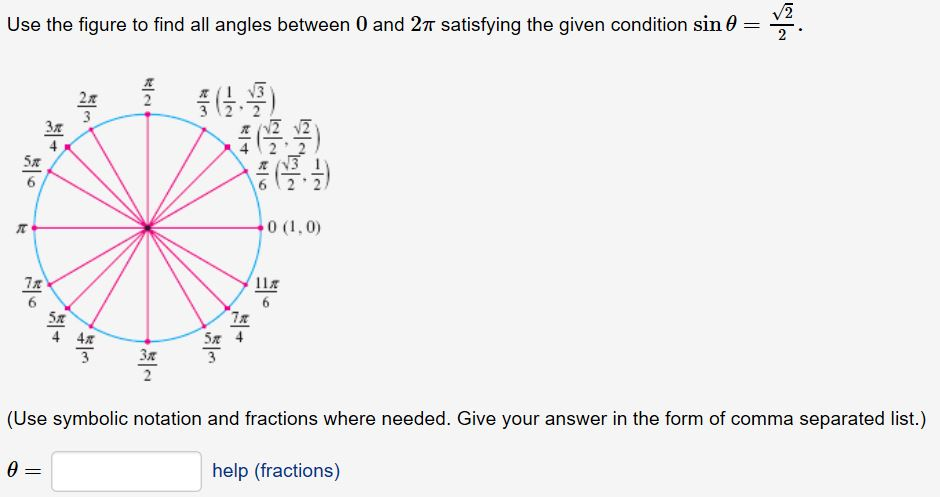# Use the figure to find all angles between 0 and 2π satisfying the given condition sin θ 3 2 2 6 2 2 7n (Use symbolic notation and fractions where needed. Give your answer in the form of comma separated list.) help (fractions)

Questionhelp_outlineImage TranscriptioncloseUse the figure to find all angles between 0 and 2π satisfying the given condition sin θ 3 2 2 6 2 2 7n (Use symbolic notation and fractions where needed. Give your answer in the form of comma separated list.) help (fractions) fullscreen

1 Rating

### Want to see this answer and more?

Experts are waiting 24/7 to provide step-by-step solutions in as fast as 30 minutes!*